## Motion in a Straight Line Questions and Answers Part-17

1. A stone is shot straight upward with a speed of 20 m/sec from a tower 200 m high. The speed with which it strikes the ground is approximately
a) 60 m/sec
b) 65 m/sec
c) 70 m/sec
d) 75 m/sec

Explanation: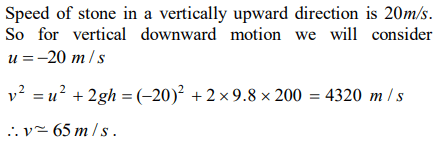2. A body freely falling from the rest has a velocity ‘v’ after it falls through a height ‘h’. The distanceit has to fall down for its velocity to become double, is
a) 2h
b) 4h
c) 6h
d) 8h

Explanation: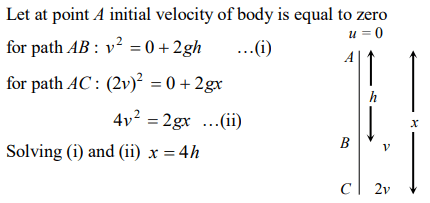3. Velocity of a body on reaching the point from which it was projected upwards, is
a) $v=0$
b) $v=2u$
c) $v=0.5u$
d) $v=u$

Explanation: Body reaches the point of projection with same velocity

4. A body projected vertically upwards with a velocity u returns to the starting point in 4 seconds. If $g=10 m\diagup sec^{2}$   , the value of u is
a) 5 m/sec
b) 10 m/sec
c) 15 m/sec
d) 20 m/sec

Explanation:5. Time taken by an object falling from rest to cover the height of $h_{1}$ and $h_{2}$ is respectively $t_{1}$ and $t_{2}$ then the ratio of $t_{1}$ to $t_{2}$ is
a) $h_{1}:h_{2}$
b) $\sqrt{h_{1}}:\sqrt{h_{2}}$
c) $h_{1}:2h_{2}$
d) $2h_{1}:h_{2}$

Explanation:6. A body is thrown vertically up from the ground. It reaches a maximum height of 100m in 5 sec. After what time it will reach the ground from the maximum height position
a) 1.2 sec
b) 5 sec
c) 10 sec
d) 25 sec

Explanation: Time of ascent = Time of descent = 5 sec

7. A body thrown vertically upwards with an initial velocity u reaches maximum height in 6 seconds. The ratio of the distances travelled by the body in the first second and the seventh second is
a) $1:1$
b) $11:1$
c) $1:2$
d) $1:11$

Explanation: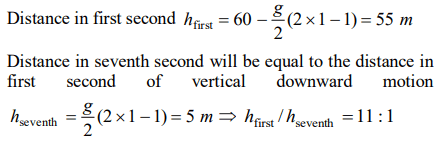8. A body, thrown upwards with some velocity, reaches the maximum height of 20m. Another body with double the mass thrown up, with double initial velocity will reach a maximum height of
a) 200 m
b) 16 m
c) 80 m
d) 40 m

Explanation: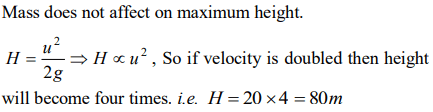9. A balloon starts rising from the ground with an acceleration of $1.25 m\diagup s^{2}$   after 8s, a stone is released from the balloon. The stone will$\left(g=10 m\diagup s^{2}\right)$
a) Reach the ground in 4 second
b) Begin to move down after being released
c) Have a displacement of 50 m
d) Cover a distance of 40 m in reaching the ground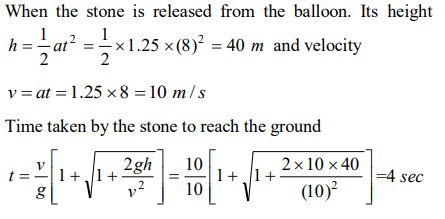d) Velocity is zero at the highest point and maximum height reached is $u^{2} \diagup 2g$